### This Flower Of Flame And Lightning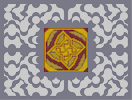Hover over the thumbnail for a full-size version.

Author invader author:invader n-art rated 2006-08-28 2006-08-28 4 by 38 people. \$This Flower Of Flame And Lightning#invader#none#=696=;9;9061906<6<:969:0780;906<0;1<0;906<078079:<:807=0:1=0:807=;=68=00:8:8:8001007=7=7=00:07<7906<6<;1<;9;9068;80;=:9007=0611190:8006=:<900;<;90;<619;<06<;<006<0;96907968179680696<0;=0:87<71;<111;<18;87=0:00;9:1111111111111=6<0087907100000000000180687:=0711000000000001180:=00;9:1000000000001=6<00<0:8;1000000000001<7=0;180611000000000001190711111110000000000011111119071100000000000118061=0;9;1000000000001=6<0:00:8:1000000000001<7=00;<0611000000000001190;<9680610000000000019079600:8;1111111111111<7=00<0;96=61=:111=:19:96<0;=0:87806879168790787=0:800:=:80:=718:=07=:=007:<;8006<0711180;9007<;=06=6807=7=:1=:8:8079:90<00;9;9;9001006<6<6<00;68;=;906<0;1<0;906<:<790690:807=0:1=0:807=0690<787<:8:8071807=7=;878;|12^396,300!12^390,294!12^384,288!12^384,282!12^384,270!12^384,264!12^390,264!12^396,264!12^402,264!12^408,264!12^402,306!12^408,312!12^408,318!12^408,324!12^408,330!12^408,336!12^402,336!12^396,336!12^390,336!12^384,336!12^390,306!12^384,312!12^378,312!12^372,312!12^366,312!12^360,312!12^360,306!12^360,300!12^360,294!12^360,288!12^402,294!12^408,288!12^414,288!12^420,288!12^426,288!12^432,288!12^432,294!12^432,300!12^432,306!12^432,312!12^408,258!12^408,252!12^408,246!12^408,240!12^402,240!12^396,240!12^390,246!12^384,252!12^378,258!12^372,264!12^438,312!12^444,312!12^450,312!12^456,312!12^456,306!12^456,300!12^450,294!12^444,288!12^438,282!12^432,276!12^384,342!12^384,348!12^384,354!12^384,360!12^390,360!12^396,360!12^402,354!12^408,348!12^414,342!12^420,336!12^354,288!12^348,288!12^342,288!12^336,288!12^336,294!12^336,300!12^342,306!12^348,312!12^354,318!12^360,324!12^372,270!12^372,276!12^366,276!12^360,276!12^354,276!12^348,276!12^342,276!12^336,276!12^426,276!12^420,276!12^420,270!12^420,264!12^420,258!12^420,252!12^420,246!12^420,240!12^366,324!12^372,330!12^372,324!12^372,336!12^372,342!12^372,348!12^372,354!12^372,360!12^420,330!12^420,324!12^426,324!12^432,324!12^438,324!12^444,324!12^450,324!12^456,324!12^414,234!12^408,228!12^402,222!12^396,216!12^390,210!12^384,204!12^384,210!12^384,216!12^384,228!12^384,222!12^384,234!12^378,240!12^372,246!12^366,252!12^462,318!12^468,312!12^474,306!12^480,300!12^486,294!12^492,288!12^486,288!12^480,288!12^474,288!12^468,288!12^462,288!12^456,282!12^450,276!12^444,270!12^378,366!12^384,372!12^390,378!12^396,384!12^402,390!12^408,396!12^408,390!12^408,384!12^408,378!12^408,372!12^408,366!12^414,360!12^420,354!12^426,348!12^330,282!12^324,288!12^318,294!12^312,300!12^306,306!12^300,312!12^306,312!12^312,312!12^318,312!12^324,312!12^330,312!12^336,318!12^342,324!12^348,330!12^360,258!12^360,264!12^354,264!12^348,264!12^342,264!12^336,264!12^336,258!12^342,252!12^342,246!12^348,240!12^348,234!12^354,228!12^354,222!12^360,216!12^366,210!12^372,204!12^378,198!12^438,264!12^432,264!12^432,258!12^432,252!12^432,246!12^432,240!12^438,240!12^444,246!12^450,246!12^456,252!12^462,252!12^468,258!12^474,258!12^480,264!12^486,270!12^492,276!12^498,282!12^432,342!12^432,336!12^438,336!12^450,336!12^444,336!12^456,336!12^456,342!12^450,348!12^450,354!12^444,360!12^444,366!12^438,372!12^438,378!12^432,384!12^426,390!12^420,396!12^414,402!12^354,336!12^360,336!12^360,342!12^360,348!12^360,354!12^360,360!12^354,360!12^348,354!12^342,354!12^336,348!12^330,348!12^324,342!12^318,342!12^312,336!12^306,330!12^300,324!12^294,318!12^468,330!12^474,324!12^480,318!12^486,312!12^492,306!12^498,300!12^504,294!12^510,288!12^516,282!12^510,276!12^504,270!12^498,264!12^492,258!12^486,252!12^480,246!12^474,246!12^468,240!12^462,240!12^456,234!12^450,234!12^444,228!12^438,228!12^432,228!12^426,228!12^420,222!12^414,216!12^408,210!12^402,204!12^396,198!12^390,192!12^384,186!12^378,180!12^372,186!12^366,192!12^360,198!12^354,204!12^348,210!12^342,216!12^342,222!12^336,228!12^336,234!12^330,240!12^330,246!12^324,252!12^324,258!12^324,264!12^324,270!12^318,276!12^312,282!12^306,288!12^300,294!12^294,300!12^288,306!12^282,312!12^276,318!12^282,324!12^288,330!12^294,336!12^300,342!12^306,348!12^312,354!12^318,354!12^324,360!12^330,360!12^336,366!12^342,366!12^348,372!12^354,372!12^360,372!12^366,372!12^372,378!12^378,384!12^384,390!12^390,396!12^396,402!12^402,408!12^408,414!12^414,420!12^420,414!12^426,408!12^432,402!12^438,396!12^444,390!12^450,384!12^450,378!12^456,372!12^456,366!12^462,360!12^462,354!12^468,348!12^468,342!12^468,336!12^528,168!12^528,174!12^528,186!12^528,180!12^528,192!12^528,198!12^528,204!12^528,210!12^528,216!12^528,222!12^528,228!12^528,240!12^528,252!12^528,246!12^528,234!12^528,258!12^528,270!12^528,264!12^528,276!12^528,282!12^528,288!12^528,294!12^528,300!12^522,192!12^516,186!12^504,174!12^504,168!12^510,180!12^516,180!12^522,186!12^522,180!12^522,174!12^522,168!12^516,168!12^510,168!12^510,174!12^516,174!12^498,168!12^492,168!12^486,168!12^480,168!12^528,306!12^528,318!12^528,312!12^528,324!12^528,330!12^528,336!12^528,342!12^528,348!12^528,354!12^528,360!12^528,372!12^528,366!12^528,378!12^528,384!12^528,390!12^528,396!12^528,402!12^528,408!12^528,414!12^528,420!12^528,426!12^528,432!12^522,432!12^516,432!12^510,432!12^522,426!12^522,420!12^522,414!12^522,408!12^516,414!12^510,420!12^504,426!12^498,432!12^504,432!12^510,426!12^516,426!12^516,420!12^492,432!12^486,432!12^480,432!12^474,432!12^468,432!12^462,432!12^456,432!12^444,432!12^450,432!12^438,432!12^432,432!12^420,432!12^426,432!12^414,432!12^408,432!12^402,432!12^396,432!12^390,432!12^384,432!12^378,432!12^372,432!12^366,432!12^360,432!12^354,432!12^348,432!12^342,432!12^336,432!12^330,432!12^324,432!12^318,432!12^312,432!12^300,432!12^300,432!12^306,432!12^294,432!12^288,432!12^282,432!12^276,432!12^270,432!12^264,432!12^264,426!12^264,420!12^264,414!12^270,426!12^270,420!12^270,414!12^270,408!12^264,408!12^264,402!12^264,396!12^276,414!12^276,420!12^276,426!12^282,426!12^288,426!12^282,420!12^264,390!12^264,378!12^264,384!12^264,372!12^264,366!12^264,360!12^264,354!12^264,348!12^264,342!12^264,336!12^264,330!12^264,324!12^264,318!12^264,312!12^264,306!12^264,300!12^264,294!12^264,288!12^264,282!12^264,276!12^264,270!12^264,264!12^264,258!12^264,252!12^264,246!12^264,240!12^264,234!12^264,228!12^264,222!12^264,216!12^264,204!12^264,210!12^264,198!12^264,192!12^264,186!12^264,174!12^264,180!12^264,168!12^270,168!12^276,168!12^282,168!12^288,168!12^282,174!12^276,180!12^270,186!12^270,192!12^276,186!12^282,180!12^288,174!12^294,168!12^270,180!12^270,174!12^276,174!12^300,168!12^306,168!12^312,168!12^318,168!12^324,168!12^330,168!12^342,168!12^336,168!12^348,168!12^360,168!12^354,168!12^366,168!12^372,168!12^378,168!12^384,168!12^390,168!12^396,168!12^402,168!12^414,168!12^402,168!12^408,168!12^420,168!12^426,168!12^438,168!12^432,168!12^444,168!12^450,168!12^456,168!12^462,168!12^468,168!12^474,168!12^282,288!12^282,282!12^282,276!12^282,270!12^282,264!12^282,258!12^282,252!12^282,246!12^282,240!12^288,234!12^288,228!12^294,222!12^294,216!12^300,210!12^300,204!12^306,198!12^312,192!12^318,186!12^324,180!12^330,180!12^336,180!12^348,180!12^342,180!12^354,180!12^348,186!12^342,192!12^336,198!12^330,204!12^324,210!12^324,216!12^318,222!12^318,228!12^312,234!12^312,240!12^306,246!12^306,252!12^300,258!12^300,264!12^294,270!12^294,276!12^288,282!12^516,258!12^516,252!12^516,246!12^516,240!12^516,234!12^516,228!12^510,222!12^504,216!12^498,210!12^492,204!12^486,204!12^480,198!12^474,198!12^468,192!12^462,192!12^456,186!12^450,186!12^444,186!12^438,186!12^432,186!12^408,186!12^414,186!12^420,186!12^426,186!12^414,192!12^420,198!12^426,198!12^432,204!12^438,204!12^444,210!12^450,210!12^456,216!12^462,216!12^468,222!12^474,222!12^480,228!12^486,228!12^492,234!12^498,240!12^504,246!12^510,252!12^438,420!12^444,420!12^450,420!12^456,420!12^462,420!12^468,420!12^474,414!12^480,408!12^486,402!12^492,396!12^492,390!12^498,384!12^498,378!12^504,372!12^504,366!12^510,360!12^510,354!12^510,348!12^510,342!12^510,336!12^510,330!12^510,324!12^510,312!12^510,318!12^504,318!12^498,324!12^498,330!12^492,336!12^492,342!12^486,348!12^486,354!12^480,360!12^480,366!12^474,372!12^474,378!12^468,384!12^468,390!12^462,396!12^456,402!12^450,408!12^444,414!12^276,342!12^276,348!12^276,354!12^276,360!12^276,366!12^276,372!12^282,378!12^288,384!12^294,390!12^300,396!12^306,396!12^312,402!12^318,402!12^324,408!12^330,408!12^336,414!12^342,414!12^348,414!12^354,414!12^360,414!12^372,414!12^366,414!12^378,414!12^384,414!12^378,408!12^372,402!12^366,402!12^360,396!12^354,396!12^348,390!12^342,390!12^336,384!12^330,384!12^324,378!12^318,378!12^312,372!12^306,372!12^300,366!12^294,360!12^288,354!12^282,348!12^384,276!0^390,270!0^390,276!0^390,282!0^390,288!0^396,288!0^396,294!0^402,288!0^402,282!0^396,282!0^396,276!0^396,270!0^402,270!0^402,276!0^402,282!0^408,270!0^408,276!0^408,282!0^420,282!0^414,282!0^426,282!0^432,282!9^510,246,0,0,22,11,1,0,0!9^510,234,0,0,23,11,1,-1,0!9^504,240,0,0,23,11,1,-1,0!9^498,234,0,0,23,11,1,-1,0!9^504,228,0,0,23,11,1,-1,0!9^492,228,0,0,23,11,1,-1,0!9^498,222,0,0,23,11,1,-1,0!9^486,222,0,0,23,12,1,-1,0!9^492,216,0,0,22,11,1,0,0!9^480,216,0,0,22,11,1,0,0!9^486,210,0,0,22,9,1,0,0!9^474,210,0,0,22,8,1,0,0!9^468,216,0,0,22,8,1,0,0!9^462,210,0,0,22,8,1,0,0!9^468,204,0,0,23,8,1,-1,0!9^480,204,0,0,23,8,1,-1,0!9^456,204,0,0,23,8,1,-1,0!9^462,198,0,0,22,8,1,0,0!9^450,198,0,0,22,8,1,0,0!9^456,192,0,0,22,8,1,0,0!9^444,204,0,0,22,8,1,0,0!9^438,198,0,0,22,8,1,0,0!9^444,192,1,0,17,6,1,0,-1!9^432,192,1,0,16,6,1,0,-1!9^420,192,1,0,16,6,1,0,-1!0^510,240!0^504,234!0^510,228!0^498,228!0^504,222!0^492,222!0^498,216!0^480,222!0^486,216!0^492,210!0^474,216!0^480,210!0^474,204!0^468,210!0^456,210!0^468,198!0^462,204!0^450,204!0^456,198!0^444,198!0^450,192!0^438,192!0^432,198!0^426,192!9^288,276,0,0,10,12,1,-1,0!9^288,264,0,0,10,11,1,-1,0!9^288,252,0,0,10,11,1,-1,0!9^294,258,0,0,10,10,1,-1,0!9^300,252,0,0,10,11,1,-1,0!9^294,246,0,0,10,10,1,-1,0!9^288,240,0,0,10,9,1,-1,0!9^300,240,0,0,9,12,1,0,0!9^294,234,0,0,10,10,1,-1,0!9^306,234,0,0,10,12,1,-1,0!9^300,228,0,0,9,12,1,0,0!9^306,222,0,0,10,12,1,-1,0!9^312,228,0,0,10,12,1,-1,0!9^300,216,0,0,10,8,1,-1,0!9^312,216,0,0,10,8,1,-1,0!9^318,210,0,0,10,8,1,-1,0!9^306,210,0,0,10,8,1,-1,0!9^312,204,0,0,10,8,1,-1,0!9^324,204,0,0,10,8,1,-1,0!9^318,198,0,0,10,8,1,-1,0!9^330,198,0,0,10,8,1,-1,0!9^324,192,0,0,10,8,1,-1,0!9^330,186,0,0,10,8,1,-1,0!9^336,192,0,0,10,8,1,-1,0!9^342,186,0,0,10,11,1,-1,0!0^336,186!0^324,186!0^330,192!0^318,192!0^312,198!0^306,204!0^324,198!0^318,204!0^312,210!0^306,216!0^300,222!0^312,222!0^318,216!0^294,228!0^306,228!0^306,240!0^300,234!0^294,240!0^288,246!0^300,246!0^294,252!0^288,258!0^294,264!0^288,270!0^438,288!0^438,294!0^438,300!0^438,306!0^444,306!0^444,306!0^450,306!0^450,300!0^444,300!0^444,294!0^414,276!0^414,270!0^414,264!0^414,258!0^414,252!0^414,246!0^414,240!0^408,234!0^402,234!0^396,234!0^390,234!0^390,222!0^390,216!0^390,228!0^396,222!0^396,234!0^396,228!0^402,228!0^390,240!0^384,240!0^384,246!0^378,246!9^504,324,0,0,22,15,1,0,0!9^504,336,0,0,22,14,1,0,0!9^498,342,0,0,22,14,1,0,0!9^504,348,0,0,22,14,1,0,0!9^498,354,0,0,22,13,1,0,0!9^492,348,0,0,22,14,1,0,0!9^504,360,0,0,22,14,1,0,0!9^492,360,0,0,23,13,1,-1,0!9^498,366,0,0,22,14,1,0,0!9^486,366,0,0,22,14,1,0,0!9^492,372,0,0,23,12,1,-1,0!9^486,378,0,0,22,14,1,0,0!9^480,372,0,0,22,14,1,0,0!9^492,384,0,0,22,16,1,0,0!9^480,384,0,0,22,16,1,0,0!9^486,390,0,0,22,15,1,0,0!9^474,390,0,0,22,15,1,0,0!9^480,396,0,0,22,15,1,0,0!9^474,402,0,0,22,16,1,0,0!9^462,402,0,0,22,16,1,0,0!9^468,396,0,0,22,16,1,0,0!9^468,408,0,0,22,16,1,0,0!9^462,414,0,0,22,16,1,0,0!9^456,408,0,0,23,16,1,-1,0!9^450,414,0,0,22,16,1,0,0!0^456,414!0^462,408!0^468,402!0^474,396!0^480,390!0^468,414!0^474,408!0^480,402!0^486,396!0^474,384!0^480,378!0^486,384!0^492,378!0^486,372!0^498,372!0^486,360!0^498,360!0^492,366!0^504,354!0^492,354!0^498,348!0^504,342!0^498,336!0^504,330!9^372,408,1,0,14,18,1,0,0!9^360,408,1,0,15,18,1,0,0!9^348,408,1,0,15,18,1,0,0!9^336,408,1,0,14,18,1,0,0!9^342,402,1,0,14,18,1,0,0!9^354,402,1,0,14,18,1,0,0!9^336,396,1,0,16,18,1,0,0!9^348,396,1,0,14,18,1,0,0!9^330,402,1,0,13,18,1,0,0!9^324,396,1,0,13,18,1,0,0!9^330,390,1,0,13,18,1,0,0!9^312,396,1,0,13,18,1,0,0!9^318,390,1,0,14,18,1,0,0!9^324,384,1,0,12,18,1,0,0!9^312,384,1,0,12,18,1,0,0!9^306,390,1,0,13,18,1,0,0!9^300,384,1,0,13,18,1,0,0!9^294,378,1,0,12,18,1,0,0!9^288,372,1,0,12,18,1,0,0!9^282,366,1,0,12,18,1,0,0!9^294,366,1,0,12,18,1,0,0!9^306,378,1,0,13,18,1,0,0!9^300,372,1,0,14,18,1,0,0!9^288,360,1,0,12,18,1,0,0!9^282,354,1,0,12,18,1,0,0!0^282,360!0^282,372!0^288,366!0^288,378!0^294,384!0^294,372!0^300,378!0^306,384!0^300,390!0^312,378!0^318,384!0^324,390!0^312,390!0^318,396!0^330,396!0^324,402!0^330,408!0^336,402!0^342,408!0^336,390!0^342,396!0^348,402!0^354,408!0^360,402!0^366,408!9^414,396,1,0,16,18,1,0,0!9^420,390,1,0,17,18,1,0,0!9^414,384,1,0,17,18,1,0,0!9^426,384,1,0,17,18,1,0,0!9^420,378,1,0,17,18,1,0,0!9^426,372,1,0,17,18,1,0,0!9^414,372,1,0,17,18,1,0,0!9^420,366,1,0,17,18,1,0,0!9^426,360,1,0,16,18,1,0,0!9^432,354,1,0,16,19,1,0,0!9^432,378,1,0,16,18,1,0,0!9^432,366,1,0,16,18,1,0,0!9^438,360,1,0,16,18,1,0,0!9^438,348,1,0,16,18,1,0,0!9^444,342,1,0,18,18,1,0,0!9^444,354,1,0,17,18,1,0,0!0^414,390!0^420,384!0^426,378!0^432,372!0^414,378!0^420,372!0^426,366!0^438,366!0^432,360!0^438,354!0^444,348!0^450,342!0^438,342!0^432,348!0^426,354!0^420,360!0^414,366!9^492,282,0,0,22,10,1,0,0!9^480,282,0,0,22,11,1,0,0!9^486,276,0,0,22,11,1,0,0!9^474,276,0,0,22,11,1,0,0!9^480,270,0,0,22,11,1,0,0!9^474,264,0,0,22,11,1,0,0!9^468,270,0,0,22,11,1,0,0!9^462,276,0,0,22,11,1,0,0!9^468,282,0,0,22,11,1,0,0!9^456,270,0,0,22,11,1,0,0!9^462,264,0,0,22,11,1,0,0!9^456,258,0,0,22,10,1,0,0!9^450,252,0,0,22,10,1,0,0!9^450,264,0,0,22,10,1,0,0!9^444,258,0,0,22,11,1,0,0!9^438,252,0,0,22,11,1,0,0!0^438,246!0^438,258!0^444,252!0^450,258!0^444,264!0^462,258!0^468,264!0^450,270!0^456,264!0^462,270!0^456,276!0^462,282!0^468,276!0^474,282!0^474,270!0^480,276!0^486,282!9^378,204,1,0,15,6,1,0,-1!9^372,210,1,0,15,6,1,0,-1!9^366,216,1,0,15,6,1,0,-1!9^360,222,1,0,15,6,1,0,-1!9^354,240,1,0,14,6,1,0,-1!9^360,234,1,0,14,6,1,0,-1!9^366,228,1,0,13,6,1,0,-1!9^372,222,1,0,14,6,1,0,-1!9^378,216,1,0,15,6,1,0,-1!9^378,228,1,0,15,6,1,0,-1!9^372,234,1,0,16,6,1,0,-1!9^366,240,1,0,16,5,1,0,-1!9^360,246,1,0,15,6,1,0,-1!9^348,246,1,0,15,6,1,0,-1!9^354,252,1,0,14,6,1,0,-1!9^348,258,1,0,15,6,1,0,-1!0^378,210!0^372,216!0^366,222!0^360,228!0^378,222!0^354,234!0^372,228!0^366,234!0^360,240!0^354,246!0^348,252!0^342,258!0^354,258!0^360,252!0^366,246!0^372,240!0^378,234!9^300,318,0,0,10,13,1,-1,0!9^312,318,0,0,10,13,1,-1,0!9^324,318,0,0,10,13,1,-1,0!9^306,324,0,0,10,14,1,-1,0!9^318,324,0,0,10,13,1,-1,0!9^330,324,0,0,10,13,1,-1,0!9^312,330,0,0,10,13,1,-1,0!9^324,330,0,0,10,13,1,-1,0!9^318,336,0,0,10,13,1,-1,0!9^330,336,0,0,10,12,1,-1,0!9^336,330,0,0,10,14,1,-1,0!9^342,336,0,0,10,13,1,-1,0!9^336,342,0,0,10,14,1,-1,0!9^348,342,0,0,10,14,1,-1,0!9^342,348,0,0,10,14,1,-1,0!9^354,348,0,0,10,14,1,-1,0!0^354,354!0^348,348!0^354,342!0^348,336!0^342,330!0^336,324!0^330,318!0^318,318!0^324,324!0^330,330!0^336,336!0^342,342!0^306,318!0^312,324!0^318,330!0^324,336!0^330,342!0^372,252!0^378,252!0^372,258!0^366,258!0^366,264!0^366,270!0^360,270!0^354,270!0^342,270!0^348,270!0^336,270!0^396,246!0^402,246!0^402,252!0^402,258!0^396,258!0^396,252!0^390,252!0^390,258!0^384,258!0^378,264!0^378,270!0^378,276!0^378,282!0^378,288!0^378,294!0^384,294!0^384,300!0^390,300!0^378,300!0^384,306!0^372,306!0^378,306!0^366,306!0^366,300!0^372,300!0^372,294!0^366,294!0^366,288!0^372,288!0^372,282!0^366,282!0^360,282!0^348,282!0^360,282!0^354,282!0^342,282!0^336,282!0^402,300!0^408,300!0^408,294!0^414,294!0^420,294!0^426,294!0^426,300!0^414,300!0^420,300!0^408,306!0^414,306!0^420,306!0^426,306!0^426,312!0^420,312!0^414,312!0^414,318!0^414,324!0^414,330!0^420,318!0^432,318!0^444,318!0^426,318!0^438,318!0^450,318!0^456,318!0^414,336!0^408,342!0^402,342!0^396,342!0^390,342!0^390,354!0^390,348!0^396,348!0^402,348!0^396,354!0^462,312!0^462,306!0^468,306!0^462,300!0^468,300!0^474,300!0^480,294!0^474,294!0^468,294!0^462,294!0^456,294!0^456,288!0^450,288!0^450,288!0^450,282!0^444,282!0^444,276!0^438,276!0^438,270!0^432,270!0^426,270!0^426,264!0^426,258!0^426,252!0^426,246!0^426,240!0^396,306!0^390,312!0^396,312!0^402,312!0^402,318!0^402,324!0^402,330!0^396,330!0^390,330!0^384,330!0^384,324!0^384,318!0^390,318!0^396,318!0^396,324!0^390,324!0^360,318!0^366,318!0^378,318!0^366,318!0^372,318!0^354,312!0^354,306!0^354,300!0^354,294!0^348,294!0^342,294!0^342,300!0^348,300!0^348,306!0^378,324!0^378,330!0^378,336!0^378,342!0^378,348!0^378,354!0^378,360!0^390,366!0^384,366!0^390,372!0^396,378!0^402,378!0^402,384!0^402,372!0^396,372!0^396,366!0^402,366!0^402,360!0^408,360!0^408,354!0^414,354!0^414,348!0^420,348!0^420,342!0^426,342!0^426,336!0^426,330!0^438,330!0^432,330!0^444,330!0^450,330!0^456,330!0^462,330!0^462,324!0^468,324!0^468,318!0^474,318!0^474,312!0^480,312!0^480,306!0^486,306!0^486,300!0^492,300!0^492,294!0^498,294!0^498,288!0^504,288!0^504,282!0^510,282!0^504,276!0^498,276!0^498,270!0^492,270!0^492,264!0^486,264!0^486,258!0^480,258!0^480,252!0^474,252!0^468,252!0^468,246!0^462,246!0^456,246!0^456,240!0^450,240!0^444,240!0^444,234!0^438,234!0^432,234!0^426,234!0^420,234!0^420,228!0^414,228!0^408,222!0^414,222!0^408,216!0^402,216!0^402,210!0^396,210!0^396,204!0^390,204!0^390,198!0^384,198!0^384,192!0^378,192!0^378,186!0^372,192!0^372,198!0^366,198!0^366,204!0^360,204!0^360,210!0^354,210!0^354,216!0^348,216!0^348,222!0^348,228!0^342,228!0^342,234!0^342,240!0^336,240!0^336,246!0^336,252!0^330,252!0^330,258!0^330,264!0^330,270!0^330,276!0^324,276!0^324,282!0^318,282!0^318,288!0^312,288!0^312,294!0^306,294!0^306,300!0^300,300!0^300,306!0^294,306!0^294,312!0^288,312!0^288,318!0^282,318!0^288,324!0^294,324!0^294,330!0^300,330!0^300,336!0^306,336!0^306,342!0^312,342!0^312,348!0^312,306!0^318,300!0^324,294!0^330,288!0^330,294!0^330,300!0^330,306!0^324,306!0^318,306!0^324,300!0^336,306!0^336,312!0^342,312!0^342,318!0^348,318!0^348,324!0^354,324!0^354,330!0^360,330!0^366,330!0^366,336!0^366,348!0^366,342!0^366,354!0^366,360!0^366,366!0^360,366!0^360,366!0^354,366!0^348,366!0^348,360!0^342,360!0^336,360!0^336,354!0^330,354!0^324,354!0^324,348!0^318,348!0^372,366!0^372,372!0^378,372!0^378,378!0^384,378!0^384,384!0^390,384!0^390,390!0^396,390!0^396,396!0^402,396!0^402,402!0^408,402!0^408,408!0^414,408!0^414,414!0^420,408!0^420,402!0^426,402!0^426,396!0^432,396!0^432,390!0^438,390!0^438,384!0^444,384!0^444,378!0^444,372!0^450,372!0^450,366!0^450,360!0^456,360!0^456,354!0^456,348!0^462,348!0^462,342!0^462,336!12^396,186!12^402,186!12^390,186!12^516,264!12^516,270!12^516,276!12^510,270!12^510,264!12^510,258!12^504,264!12^504,258!12^504,252!12^498,258!12^498,252!12^498,246!12^492,252!12^492,246!12^492,240!12^486,246!12^486,240!12^486,234!12^480,234!12^480,240!12^474,240!12^474,234!12^474,228!12^468,228!12^468,234!12^462,234!12^462,228!12^462,222!12^456,222!12^456,228!12^450,228!12^450,222!12^450,216!12^444,222!12^444,216!12^438,222!12^438,216!12^438,210!12^432,210!12^432,216!12^432,222!12^426,222!12^426,216!12^426,210!12^426,204!12^420,204!12^420,210!12^420,216!12^414,210!12^414,204!12^414,198!12^408,204!12^408,198!12^408,192!12^402,192!12^396,192!12^402,198!12^384,180!12^390,180!12^396,180!12^402,180!12^408,180!12^414,180!12^420,180!12^432,180!12^426,180!12^438,180!12^444,180!12^372,180!12^366,180!12^360,180!12^360,186!12^366,186!12^354,186!12^360,192!12^354,192!12^348,192!12^354,198!12^348,198!12^342,198!12^348,204!12^342,204!12^336,204!12^342,210!12^336,210!12^330,210!12^330,216!12^336,216!12^336,222!12^330,222!12^324,222!12^324,228!12^330,228!12^324,234!12^330,234!12^318,234!12^318,240!12^330,240!12^324,240!12^324,246!12^318,246!12^312,246!12^312,252!12^318,252!12^318,258!12^312,258!12^306,258!12^306,264!12^312,264!12^318,264!12^312,270!12^318,270!12^306,270!12^300,270!12^300,276!12^312,276!12^306,276!12^306,282!12^300,282!12^294,282!12^300,288!12^294,288!12^288,288!12^294,294!12^276,252!12^276,258!12^276,264!12^276,270!12^276,276!12^276,282!12^276,288!12^276,294!12^276,300!12^276,306!12^276,312!12^282,306!12^282,300!12^282,288!12^282,294!12^288,294!12^288,300!12^276,324!12^276,336!12^276,330!12^408,420!12^402,420!12^396,420!12^390,420!12^384,420!12^378,420!12^372,420!12^366,420!12^360,420!12^354,420!12^348,420!12^330,414!12^342,420!12^402,414!12^396,414!12^390,414!12^396,408!12^390,408!12^384,408!12^390,402!12^384,402!12^378,402!12^384,396!12^378,396!12^378,396!12^372,396!12^366,396!12^378,390!12^372,390!12^366,390!12^360,390!12^354,390!12^372,384!12^366,384!12^360,384!12^354,384!12^348,384!12^342,384!12^366,378!12^360,378!12^354,378!12^348,378!12^342,378!12^336,378!12^330,378!12^342,372!12^336,372!12^330,372!12^324,372!12^318,372!12^330,366!12^324,366!12^318,366!12^306,366!12^312,366!12^318,360!12^312,360!12^306,360!12^300,360!12^306,354!12^300,354!12^294,354!12^300,348!12^294,348!12^288,348!12^294,342!12^288,342!12^282,342!12^288,336!12^282,336!12^282,330!12^420,420!12^426,420!12^432,420!12^516,288!12^516,294!12^516,300!12^516,306!12^516,312!12^516,318!12^516,324!12^516,330!12^516,336!12^516,342!12^516,348!12^510,294!12^510,300!12^510,306!12^504,300!12^504,306!12^504,312!12^498,318!12^498,312!12^498,306!12^492,312!12^492,318!12^492,324!12^492,330!12^486,318!12^486,324!12^486,330!12^486,336!12^486,342!12^480,324!12^480,330!12^480,336!12^480,342!12^480,348!12^480,354!12^474,330!12^474,336!12^474,348!12^474,342!12^474,354!12^474,360!12^474,366!12^468,354!12^468,360!12^468,366!12^468,372!12^468,378!12^462,366!12^462,372!12^462,384!12^462,378!12^462,390!12^456,378!12^456,384!12^456,390!12^456,396!12^450,390!12^450,396!12^450,402!12^444,396!12^444,402!12^444,408!12^438,402!12^438,408!12^438,414!12^432,408!12^432,414!12^426,414!0^474,426!0^474,420!0^480,426!0^480,420!0^480,414!0^492,414!0^486,414!0^498,414!0^504,414!0^510,414!0^498,420!0^504,420!0^492,420!0^486,420!0^486,426!0^492,426!0^498,426!0^486,408!0^492,408!0^498,408!0^504,408!0^510,408!0^516,408!0^492,402!0^498,402!0^504,402!0^510,402!0^516,402!0^522,402!0^522,390!0^522,396!0^510,396!0^516,390!0^510,390!0^516,396!0^504,396!0^498,396!0^498,390!0^504,390!0^504,384!0^510,384!0^516,384!0^522,384!0^522,378!0^516,378!0^504,378!0^510,378!0^510,372!0^516,372!0^522,372!0^522,366!0^516,366!0^510,366!0^516,360!0^522,360!0^522,354!0^516,354!0^522,348!0^522,342!0^522,336!0^522,330!0^522,324!0^522,312!0^522,318!0^522,306!0^522,300!0^522,294!0^522,288!0^522,282!0^522,276!0^522,270!0^522,264!0^522,258!0^522,252!0^522,246!0^522,240!0^522,234!0^522,228!0^522,222!0^516,222!0^522,216!0^516,216!0^510,216!0^510,210!0^504,210!0^516,210!0^522,210!0^522,204!0^516,204!0^510,210!0^510,204!0^498,204!0^504,204!0^504,198!0^504,192!0^504,186!0^504,180!0^510,186!0^510,192!0^510,198!0^516,198!0^522,198!0^516,192!0^498,198!0^486,198!0^492,198!0^498,192!0^498,186!0^498,180!0^498,174!0^492,174!0^492,180!0^492,186!0^492,192!0^486,192!0^480,192!0^474,192!0^486,186!0^480,186!0^474,186!0^468,186!0^462,186!0^486,186!0^486,180!0^480,180!0^474,180!0^462,180!0^468,180!0^456,180!0^450,180!0^486,174!0^480,174!0^474,174!0^468,174!0^462,174!0^450,174!0^456,174!0^444,174!0^438,174!0^432,174!0^414,174!0^420,174!0^426,174!0^408,174!0^402,174!0^396,174!0^390,174!0^384,174!0^378,174!0^372,174!0^366,174!0^354,174!0^348,174!0^360,174!0^342,174!0^336,174!0^330,174!0^324,174!0^318,174!0^312,174!0^306,174!0^300,174!0^294,174!0^318,180!0^312,180!0^306,180!0^300,180!0^294,180!0^288,180!0^282,186!0^288,186!0^294,186!0^300,186!0^306,186!0^312,186!0^270,198!0^276,198!0^282,198!0^276,192!0^282,192!0^288,192!0^294,192!0^300,192!0^306,192!0^294,198!0^288,198!0^300,198!0^294,204!0^288,210!0^288,204!0^282,204!0^276,204!0^270,204!0^294,210!0^282,210!0^276,210!0^270,210!0^276,216!0^270,216!0^282,216!0^288,216!0^288,222!0^282,222!0^276,222!0^270,222!0^270,228!0^276,228!0^282,228!0^282,234!0^276,234!0^270,234!0^270,240!0^276,240!0^276,246!0^270,246!0^270,252!0^270,258!0^270,264!0^270,264!0^270,270!0^270,276!0^270,282!0^270,288!0^270,294!0^270,306!0^270,300!0^270,312!0^270,318!0^270,324!0^270,330!0^270,336!0^270,342!0^270,348!0^270,354!0^270,360!0^270,366!0^270,372!0^270,378!0^270,384!0^270,390!0^270,396!0^270,402!0^276,378!0^276,384!0^276,396!0^276,384!0^276,390!0^276,402!0^276,408!0^282,384!0^282,390!0^282,396!0^282,402!0^282,408!0^282,414!0^288,390!0^288,396!0^288,402!0^288,408!0^288,414!0^288,420!0^294,396!0^294,408!0^294,402!0^294,414!0^294,420!0^294,426!0^300,402!0^306,402!0^306,408!0^300,408!0^300,414!0^306,414!0^306,420!0^300,420!0^300,426!0^306,426!0^318,408!0^312,408!0^324,414!0^318,414!0^312,414!0^312,420!0^318,420!0^324,420!0^336,420!0^330,420!0^312,426!0^318,426!0^324,426!0^330,426!0^336,426!0^342,426!0^348,426!0^354,426!0^360,426!0^366,426!0^372,426!0^378,426!0^390,426!0^384,426!0^396,426!0^402,426!0^408,426!0^414,426!0^420,426!0^426,426!0^432,426!0^438,426!0^444,426!0^450,426!0^456,426!0^462,426!0^468,426# Another one of these I made, but better than the last. It took me a long time. Please rate & comment.

## Other maps by this author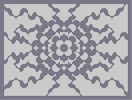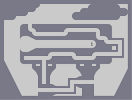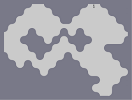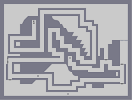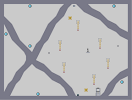An Architects Kaleidoscope Nemesis Castle Wibble-wobble wibble-wobble jelly on a plate Whatever happened to physics? Passage ways Worm Colony

Pages: (0)

### this is

kinda ugly, but meh. 3

i don't understand what you were talking about: http://numa.notdot.net/map/59303
4.5

### Wow ...

Your map is really good ! 5/5 ;)
I didn't actually have your tileset in mind when I made this, but I can see the similarities. And anyway, I have made tilesets of this style before. Look at A Golden Brilliance for instance. I also have another one lying aroun that I made ages ago and never submitted.

### You really can't say that

because yours probably isn't the first of those anyway, there may be similarities, but there are most likely similarities from that to something that came before it, so he doesn't need to credit you, if anything everyone should credit the first guy to make something with tiles like that, so meh.

### ummmm,

im pretty sure you ripped off my tileset to make this...

im dissapointed that you didnt credit me, can someone please look at the similarities because i deserve a little bit of credit http://numa.notdot.net/map/50728

you even commented on the map, so its not like youve never seen it before

dont take me the wrong way, im just a bit put out by not mentioning my tileset

i was prepared to rate a 5 before i realised how similar they were...

### Damn

Knocked off top rated. Ah well, it was good while it lasted, and I'll do it again, I'm making a bigger one...

### Awesome!

great art dude. 4.5/5

### Damn

Made a mistake, the tileset is not symmetrical, but I can't change it. It should be this:

=696=;9;9061906<6<:969:0780;906<0;1<0;906<078079:<:807=0:1=0:807=;=68=00:8:8:8001007=7=7=00:07<7906<6<;1<;9;9068;80;=:9007=0611190:8006=:<900;<;90;<619;<06<;<006<0;96907968179680696<0;=0:87<71<;111<;18;87=0:00;9:1111111111111=6<0087907100000000000180687:=0711000000000001180:=00;9:1000000000001=6<00<0:8;1000000000001<7=0;180611000000000001190711111110000000000011111119071100000000000118061=0;9:1000000000001=6<0:00:8;1000000000001<7=00;<0611000000000001190;<9680610000000000019079600:8;1111111111111<7=00<0;96=61=:111=:19:96<0;=0:87806879168790787=0:800:=:80:=718:=07=:=007:<;8006<0711180;9007<;=06=6807=7=:1=:8:8079:90<00;9;9;9001006<6<6<00;68;=;906<0;1<0;906<:<790690:807=0:1=0:807=0690<787<:8:8071807=7=;878;|12^396,300!12^390,294!12^384,288!12^384,282!12^384,270!12^384,264!12^390,264!12^396,264!12^402,264!12^408,264!12^402,306!12^408,312!12^408,318!12^408,324!12^408,330!12^408,336!12^402,336!12^396,336!12^390,336!12^384,336!12^390,306!12^384,312!12^378,312!12^372,312!12^366,312!12^360,312!12^360,306!12^360,300!12^360,294!12^360,288!12^402,294!12^408,288!12^414,288!12^420,288!12^426,288!12^432,288!12^432,294!12^432,300!12^432,306!12^432,312!12^408,258!12^408,252!12^408,246!12^408,240!12^402,240!12^396,240!12^390,246!12^384,252!12^378,258!12^372,264!12^438,312!12^444,312!12^450,312!12^456,312!12^456,306!12^456,300!12^450,294!12^444,288!12^438,282!12^432,276!12^384,342!12^384,348!12^384,354!12^384,360!12^390,360!12^396,360!12^402,354!12^408,348!12^414,342!12^420,336!12^354,288!12^348,288!12^342,288!12^336,288!12^336,294!12^336,300!12^342,306!12^348,312!12^354,318!12^360,324!12^372,270!12^372,276!12^366,276!12^360,276!12^354,276!12^348,276!12^342,276!12^336,276!12^426,276!12^420,276!12^420,270!12^420,264!12^420,258!12^420,252!12^420,246!12^420,240!12^366,324!12^372,330!12^372,324!12^372,336!12^372,342!12^372,348!12^372,354!12^372,360!12^420,330!12^420,324!12^426,324!12^432,324!12^438,324!12^444,324!12^450,324!12^456,324!12^414,234!12^408,228!12^402,222!12^396,216!12^390,210!12^384,204!12^384,210!12^384,216!12^384,228!12^384,222!12^384,234!12^378,240!12^372,246!12^366,252!12^462,318!12^468,312!12^474,306!12^480,300!12^486,294!12^492,288!12^486,288!12^480,288!12^474,288!12^468,288!12^462,288!12^456,282!12^450,276!12^444,270!12^378,366!12^384,372!12^390,378!12^396,384!12^402,390!12^408,396!12^408,390!12^408,384!12^408,378!12^408,372!12^408,366!12^414,360!12^420,354!12^426,348!12^330,282!12^324,288!12^318,294!12^312,300!12^306,306!12^300,312!12^306,312!12^312,312!12^318,312!12^324,312!12^330,312!12^336,318!12^342,324!12^348,330!12^360,258!12^360,264!12^354,264!12^348,264!12^342,264!12^336,264!12^336,258!12^342,252!12^342,246!12^348,240!12^348,234!12^354,228!12^354,222!12^360,216!12^366,210!12^372,204!12^378,198!12^438,264!12^432,264!12^432,258!12^432,252!12^432,246!12^432,240!12^438,240!12^444,246!12^450,246!12^456,252!12^462,252!12^468,258!12^474,258!12^480,264!12^486,270!12^492,276!12^498,282!12^432,342!12^432,336!12^438,336!12^450,336!12^444,336!12^456,336!12^456,342!12^450,348!12^450,354!12^444,360!12^444,366!12^438,372!12^438,378!12^432,384!12^426,390!12^420,396!12^414,402!12^354,336!12^360,336!12^360,342!12^360,348!12^360,354!12^360,360!12^354,360!12^348,354!12^342,354!12^336,348!12^330,348!12^324,342!12^318,342!12^312,336!12^306,330!12^300,324!12^294,318!12^468,330!12^474,324!12^480,318!12^486,312!12^492,306!12^498,300!12^504,294!12^510,288!12^516,282!12^510,276!12^504,270!12^498,264!12^492,258!12^486,252!12^480,246!12^474,246!12^468,240!12^462,240!12^456,234!12^450,234!12^444,228!12^438,228!12^432,228!12^426,228!12^420,222!12^414,216!12^408,210!12^402,204!12^396,198!12^390,192!12^384,186!12^378,180!12^372,186!12^366,192!12^360,198!12^354,204!12^348,210!12^342,216!12^342,222!12^336,228!12^336,234!12^330,240!12^330,246!12^324,252!12^324,258!12^324,264!12^324,270!12^318,276!12^312,282!12^306,288!12^300,294!12^294,300!12^288,306!12^282,312!12^276,318!12^282,324!12^288,330!12^294,336!12^300,342!12^306,348!12^312,354!12^318,354!12^324,360!12^330,360!12^336,366!12^342,366!12^348,372!12^354,372!12^360,372!12^366,372!12^372,378!12^378,384!12^384,390!12^390,396!12^396,402!12^402,408!12^408,414!12^414,420!12^420,414!12^426,408!12^432,402!12^438,396!12^444,390!12^450,384!12^450,378!12^456,372!12^456,366!12^462,360!12^462,354!12^468,348!12^468,342!12^468,336!12^528,168!12^528,174!12^528,186!12^528,180!12^528,192!12^528,198!12^528,204!12^528,210!12^528,216!12^528,222!12^528,228!12^528,240!12^528,252!12^528,246!12^528,234!12^528,258!12^528,270!12^528,264!12^528,276!12^528,282!12^528,288!12^528,294!12^528,300!12^522,192!12^516,186!12^504,174!12^504,168!12^510,180!12^516,180!12^522,186!12^522,180!12^522,174!12^522,168!12^516,168!12^510,168!12^510,174!12^516,174!12^498,168!12^492,168!12^486,168!12^480,168!12^528,306!12^528,318!12^528,312!12^528,324!12^528,330!12^528,336!12^528,342!12^528,348!12^528,354!12^528,360!12^528,372!12^528,366!12^528,378!12^528,384!12^528,390!12^528,396!12^528,402!12^528,408!12^528,414!12^528,420!12^528,426!12^528,432!12^522,432!12^516,432!12^510,432!12^522,426!12^522,420!12^522,414!12^522,408!12^516,414!12^510,420!12^504,426!12^498,432!12^504,432!12^510,426!12^516,426!12^516,420!12^492,432!12^486,432!12^480,432!12^474,432!12^468,432!12^462,432!12^456,432!12^444,432!12^450,432!12^438,432!12^432,432!12^420,432!12^426,432!12^414,432!12^408,432!12^402,432!12^396,432!12^390,432!12^384,432!12^378,432!12^372,432!12^366,432!12^360,432!12^354,432!12^348,432!12^342,432!12^336,432!12^330,432!12^324,432!12^318,432!12^312,432!12^300,432!12^300,432!12^306,432!12^294,432!12^288,432!12^282,432!12^276,432!12^270,432!12^264,432!12^264,426!12^264,420!12^264,414!12^270,426!12^270,420!12^270,414!12^270,408!12^264,408!12^264,402!12^264,396!12^276,414!12^276,420!12^276,426!12^282,426!12^288,426!12^282,420!12^264,390!12^264,378!12^264,384!12^264,372!12^264,366!12^264,360!12^264,354!12^264,348!12^264,342!12^264,336!12^264,330!12^264,324!12^264,318!12^264,312!12^264,306!12^264,300!12^264,294!12^264,288!12^264,282!12^264,276!12^264,270!12^264,264!12^264,258!12^264,252!12^264,246!12^264,240!12^264,234!12^264,228!12^264,222!12^264,216!12^264,204!12^264,210!12^264,198!12^264,192!12^264,186!12^264,174!12^264,180!12^264,168!12^270,168!12^276,168!12^282,168!12^288,168!12^282,174!12^276,180!12^270,186!12^270,192!12^276,186!12^282,180!12^288,174!12^294,168!12^270,180!12^270,174!12^276,174!12^300,168!12^306,168!12^312,168!12^318,168!12^324,168!12^330,168!12^342,168!12^336,168!12^348,168!12^360,168!12^354,168!12^366,168!12^372,168!12^378,168!12^384,168!12^390,168!12^396,168!12^402,168!12^414,168!12^402,168!12^408,168!12^420,168!12^426,168!12^438,168!12^432,168!12^444,168!12^450,168!12^456,168!12^462,168!12^468,168!12^474,168!12^282,288!12^282,282!12^282,276!12^282,270!12^282,264!12^282,258!12^282,252!12^282,246!12^282,240!12^288,234!12^288,228!12^294,222!12^294,216!12^300,210!12^300,204!12^306,198!12^312,192!12^318,186!12^324,180!12^330,180!12^336,180!12^348,180!12^342,180!12^354,180!12^348,186!12^342,192!12^336,198!12^330,204!12^324,210!12^324,216!12^318,222!12^318,228!12^312,234!12^312,240!12^306,246!12^306,252!12^300,258!12^300,264!12^294,270!12^294,276!12^288,282!12^516,258!12^516,252!12^516,246!12^516,240!12^516,234!12^516,228!12^510,222!12^504,216!12^498,210!12^492,204!12^486,204!12^480,198!12^474,198!12^468,192!12^462,192!12^456,186!12^450,186!12^444,186!12^438,186!12^432,186!12^408,186!12^414,186!12^420,186!12^426,186!12^414,192!12^420,198!12^426,198!12^432,204!12^438,204!12^444,210!12^450,210!12^456,216!12^462,216!12^468,222!12^474,222!12^480,228!12^486,228!12^492,234!12^498,240!12^504,246!12^510,252!12^438,420!12^444,420!12^450,420!12^456,420!12^462,420!12^468,420!12^474,414!12^480,408!12^486,402!12^492,396!12^492,390!12^498,384!12^498,378!12^504,372!12^504,366!12^510,360!12^510,354!12^510,348!12^510,342!12^510,336!12^510,330!12^510,324!12^510,312!12^510,318!12^504,318!12^498,324!12^498,330!12^492,336!12^492,342!12^486,348!12^486,354!12^480,360!12^480,366!12^474,372!12^474,378!12^468,384!12^468,390!12^462,396!12^456,402!12^450,408!12^444,414!12^276,342!12^276,348!12^276,354!12^276,360!12^276,366!12^276,372!12^282,378!12^288,384!12^294,390!12^300,396!12^306,396!12^312,402!12^318,402!12^324,408!12^330,408!12^336,414!12^342,414!12^348,414!12^354,414!12^360,414!12^372,414!12^366,414!12^378,414!12^384,414!12^378,408!12^372,402!12^366,402!12^360,396!12^354,396!12^348,390!12^342,390!12^336,384!12^330,384!12^324,378!12^318,378!12^312,372!12^306,372!12^300,366!12^294,360!12^288,354!12^282,348!12^384,276!0^390,270!0^390,276!0^390,282!0^390,288!0^396,288!0^396,294!0^402,288!0^402,282!0^396,282!0^396,276!0^396,270!0^402,270!0^402,276!0^402,282!0^408,270!0^408,276!0^408,282!0^420,282!0^414,282!0^426,282!0^432,282!9^510,246,0,0,22,11,1,0,0!9^510,234,0,0,23,11,1,-1,0!9^504,240,0,0,23,11,1,-1,0!9^498,234,0,0,23,11,1,-1,0!9^504,228,0,0,23,11,1,-1,0!9^492,228,0,0,23,11,1,-1,0!9^498,222,0,0,23,11,1,-1,0!9^486,222,0,0,23,12,1,-1,0!9^492,216,0,0,22,11,1,0,0!9^480,216,0,0,22,11,1,0,0!9^486,210,0,0,22,9,1,0,0!9^474,210,0,0,22,8,1,0,0!9^468,216,0,0,22,8,1,0,0!9^462,210,0,0,22,8,1,0,0!9^468,204,0,0,23,8,1,-1,0!9^480,204,0,0,23,8,1,-1,0!9^456,204,0,0,23,8,1,-1,0!9^462,198,0,0,22,8,1,0,0!9^450,198,0,0,22,8,1,0,0!9^456,192,0,0,22,8,1,0,0!9^444,204,0,0,22,8,1,0,0!9^438,198,0,0,22,8,1,0,0!9^444,192,1,0,17,6,1,0,-1!9^432,192,1,0,16,6,1,0,-1!9^420,192,1,0,16,6,1,0,-1!0^510,240!0^504,234!0^510,228!0^498,228!0^504,222!0^492,222!0^498,216!0^480,222!0^486,216!0^492,210!0^474,216!0^480,210!0^474,204!0^468,210!0^456,210!0^468,198!0^462,204!0^450,204!0^456,198!0^444,198!0^450,192!0^438,192!0^432,198!0^426,192!9^288,276,0,0,10,12,1,-1,0!9^288,264,0,0,10,11,1,-1,0!9^288,252,0,0,10,11,1,-1,0!9^294,258,0,0,10,10,1,-1,0!9^300,252,0,0,10,11,1,-1,0!9^294,246,0,0,10,10,1,-1,0!9^288,240,0,0,10,9,1,-1,0!9^300,240,0,0,9,12,1,0,0!9^294,234,0,0,10,10,1,-1,0!9^306,234,0,0,10,12,1,-1,0!9^300,228,0,0,9,12,1,0,0!9^306,222,0,0,10,12,1,-1,0!9^312,228,0,0,10,12,1,-1,0!9^300,216,0,0,10,8,1,-1,0!9^312,216,0,0,10,8,1,-1,0!9^318,210,0,0,10,8,1,-1,0!9^306,210,0,0,10,8,1,-1,0!9^312,204,0,0,10,8,1,-1,0!9^324,204,0,0,10,8,1,-1,0!9^318,198,0,0,10,8,1,-1,0!9^330,198,0,0,10,8,1,-1,0!9^324,192,0,0,10,8,1,-1,0!9^330,186,0,0,10,8,1,-1,0!9^336,192,0,0,10,8,1,-1,0!9^342,186,0,0,10,11,1,-1,0!0^336,186!0^324,186!0^330,192!0^318,192!0^312,198!0^306,204!0^324,198!0^318,204!0^312,210!0^306,216!0^300,222!0^312,222!0^318,216!0^294,228!0^306,228!0^306,240!0^300,234!0^294,240!0^288,246!0^300,246!0^294,252!0^288,258!0^294,264!0^288,270!0^438,288!0^438,294!0^438,300!0^438,306!0^444,306!0^444,306!0^450,306!0^450,300!0^444,300!0^444,294!0^414,276!0^414,270!0^414,264!0^414,258!0^414,252!0^414,246!0^414,240!0^408,234!0^402,234!0^396,234!0^390,234!0^390,222!0^390,216!0^390,228!0^396,222!0^396,234!0^396,228!0^402,228!0^390,240!0^384,240!0^384,246!0^378,246!9^504,324,0,0,22,15,1,0,0!9^504,336,0,0,22,14,1,0,0!9^498,342,0,0,22,14,1,0,0!9^504,348,0,0,22,14,1,0,0!9^498,354,0,0,22,13,1,0,0!9^492,348,0,0,22,14,1,0,0!9^504,360,0,0,22,14,1,0,0!9^492,360,0,0,23,13,1,-1,0!9^498,366,0,0,22,14,1,0,0!9^486,366,0,0,22,14,1,0,0!9^492,372,0,0,23,12,1,-1,0!9^486,378,0,0,22,14,1,0,0!9^480,372,0,0,22,14,1,0,0!9^492,384,0,0,22,16,1,0,0!9^480,384,0,0,22,16,1,0,0!9^486,390,0,0,22,15,1,0,0!9^474,390,0,0,22,15,1,0,0!9^480,396,0,0,22,15,1,0,0!9^474,402,0,0,22,16,1,0,0!9^462,402,0,0,22,16,1,0,0!9^468,396,0,0,22,16,1,0,0!9^468,408,0,0,22,16,1,0,0!9^462,414,0,0,22,16,1,0,0!9^456,408,0,0,23,16,1,-1,0!9^450,414,0,0,22,16,1,0,0!0^456,414!0^462,408!0^468,402!0^474,396!0^480,390!0^468,414!0^474,408!0^480,402!0^486,396!0^474,384!0^480,378!0^486,384!0^492,378!0^486,372!0^498,372!0^486,360!0^498,360!0^492,366!0^504,354!0^492,354!0^498,348!0^504,342!0^498,336!0^504,330!9^372,408,1,0,14,18,1,0,0!9^360,408,1,0,15,18,1,0,0!9^348,408,1,0,15,18,1,0,0!9^336,408,1,0,14,18,1,0,0!9^342,402,1,0,14,18,1,0,0!9^354,402,1,0,14,18,1,0,0!9^336,396,1,0,16,18,1,0,0!9^348,396,1,0,14,18,1,0,0!9^330,402,1,0,13,18,1,0,0!9^324,396,1,0,13,18,1,0,0!9^330,390,1,0,13,18,1,0,0!9^312,396,1,0,13,18,1,0,0!9^318,390,1,0,14,18,1,0,0!9^324,384,1,0,12,18,1,0,0!9^312,384,1,0,12,18,1,0,0!9^306,390,1,0,13,18,1,0,0!9^300,384,1,0,13,18,1,0,0!9^294,378,1,0,12,18,1,0,0!9^288,372,1,0,12,18,1,0,0!9^282,366,1,0,12,18,1,0,0!9^294,366,1,0,12,18,1,0,0!9^306,378,1,0,13,18,1,0,0!9^300,372,1,0,14,18,1,0,0!9^288,360,1,0,12,18,1,0,0!9^282,354,1,0,12,18,1,0,0!0^282,360!0^282,372!0^288,366!0^288,378!0^294,384!0^294,372!0^300,378!0^306,384!0^300,390!0^312,378!0^318,384!0^324,390!0^312,390!0^318,396!0^330,396!0^324,402!0^330,408!0^336,402!0^342,408!0^336,390!0^342,396!0^348,402!0^354,408!0^360,402!0^366,408!9^414,396,1,0,16,18,1,0,0!9^420,390,1,0,17,18,1,0,0!9^414,384,1,0,17,18,1,0,0!9^426,384,1,0,17,18,1,0,0!9^420,378,1,0,17,18,1,0,0!9^426,372,1,0,17,18,1,0,0!9^414,372,1,0,17,18,1,0,0!9^420,366,1,0,17,18,1,0,0!9^426,360,1,0,16,18,1,0,0!9^432,354,1,0,16,19,1,0,0!9^432,378,1,0,16,18,1,0,0!9^432,366,1,0,16,18,1,0,0!9^438,360,1,0,16,18,1,0,0!9^438,348,1,0,16,18,1,0,0!9^444,342,1,0,18,18,1,0,0!9^444,354,1,0,17,18,1,0,0!0^414,390!0^420,384!0^426,378!0^432,372!0^414,378!0^420,372!0^426,366!0^438,366!0^432,360!0^438,354!0^444,348!0^450,342!0^438,342!0^432,348!0^426,354!0^420,360!0^414,366!9^492,282,0,0,22,10,1,0,0!9^480,282,0,0,22,11,1,0,0!9^486,276,0,0,22,11,1,0,0!9^474,276,0,0,22,11,1,0,0!9^480,270,0,0,22,11,1,0,0!9^474,264,0,0,22,11,1,0,0!9^468,270,0,0,22,11,1,0,0!9^462,276,0,0,22,11,1,0,0!9^468,282,0,0,22,11,1,0,0!9^456,270,0,0,22,11,1,0,0!9^462,264,0,0,22,11,1,0,0!9^456,258,0,0,22,10,1,0,0!9^450,252,0,0,22,10,1,0,0!9^450,264,0,0,22,10,1,0,0!9^444,258,0,0,22,11,1,0,0!9^438,252,0,0,22,11,1,0,0!0^438,246!0^438,258!0^444,252!0^450,258!0^444,264!0^462,258!0^468,264!0^450,270!0^456,264!0^462,270!0^456,276!0^462,282!0^468,276!0^474,282!0^474,270!0^480,276!0^486,282!9^378,204,1,0,15,6,1,0,-1!9^372,210,1,0,15,6,1,0,-1!9^366,216,1,0,15,6,1,0,-1!9^360,222,1,0,15,6,1,0,-1!9^354,240,1,0,14,6,1,0,-1!9^360,234,1,0,14,6,1,0,-1!9^366,228,1,0,13,6,1,0,-1!9^372,222,1,0,14,6,1,0,-1!9^378,216,1,0,15,6,1,0,-1!9^378,228,1,0,15,6,1,0,-1!9^372,234,1,0,16,6,1,0,-1!9^366,240,1,0,16,5,1,0,-1!9^360,246,1,0,15,6,1,0,-1!9^348,246,1,0,15,6,1,0,-1!9^354,252,1,0,14,6,1,0,-1!9^348,258,1,0,15,6,1,0,-1!0^378,210!0^372,216!0^366,222!0^360,228!0^378,222!0^354,234!0^372,228!0^366,234!0^360,240!0^354,246!0^348,252!0^342,258!0^354,258!0^360,252!0^366,246!0^372,240!0^378,234!9^300,318,0,0,10,13,1,-1,0!9^312,318,0,0,10,13,1,-1,0!9^324,318,0,0,10,13,1,-1,0!9^306,324,0,0,10,14,1,-1,0!9^318,324,0,0,10,13,1,-1,0!9^330,324,0,0,10,13,1,-1,0!9^312,330,0,0,10,13,1,-1,0!9^324,330,0,0,10,13,1,-1,0!9^318,336,0,0,10,13,1,-1,0!9^330,336,0,0,10,12,1,-1,0!9^336,330,0,0,10,14,1,-1,0!9^342,336,0,0,10,13,1,-1,0!9^336,342,0,0,10,14,1,-1,0!9^348,342,0,0,10,14,1,-1,0!9^342,348,0,0,10,14,1,-1,0!9^354,348,0,0,10,14,1,-1,0!0^354,354!0^348,348!0^354,342!0^348,336!0^342,330!0^336,324!0^330,318!0^318,318!0^324,324!0^330,330!0^336,336!0^342,342!0^306,318!0^312,324!0^318,330!0^324,336!0^330,342!0^372,252!0^378,252!0^372,258!0^366,258!0^366,264!0^366,270!0^360,270!0^354,270!0^342,270!0^348,270!0^336,270!0^396,246!0^402,246!0^402,252!0^402,258!0^396,258!0^396,252!0^390,252!0^390,258!0^384,258!0^378,264!0^378,270!0^378,276!0^378,282!0^378,288!0^378,294!0^384,294!0^384,300!0^390,300!0^378,300!0^384,306!0^372,306!0^378,306!0^366,306!0^366,300!0^372,300!0^372,294!0^366,294!0^366,288!0^372,288!0^372,282!0^366,282!0^360,282!0^348,282!0^360,282!0^354,282!0^342,282!0^336,282!0^402,300!0^408,300!0^408,294!0^414,294!0^420,294!0^426,294!0^426,300!0^414,300!0^420,300!0^408,306!0^414,306!0^420,306!0^426,306!0^426,312!0^420,312!0^414,312!0^414,318!0^414,324!0^414,330!0^420,318!0^432,318!0^444,318!0^426,318!0^438,318!0^450,318!0^456,318!0^414,336!0^408,342!0^402,342!0^396,342!0^390,342!0^390,354!0^390,348!0^396,348!0^402,348!0^396,354!0^462,312!0^462,306!0^468,306!0^462,300!0^468,300!0^474,300!0^480,294!0^474,294!0^468,294!0^462,294!0^456,294!0^456,288!0^450,288!0^450,288!0^450,282!0^444,282!0^444,276!0^438,276!0^438,270!0^432,270!0^426,270!0^426,264!0^426,258!0^426,252!0^426,246!0^426,240!0^396,306!0^390,312!0^396,312!0^402,312!0^402,318!0^402,324!0^402,330!0^396,330!0^390,330!0^384,330!0^384,324!0^384,318!0^390,318!0^396,318!0^396,324!0^390,324!0^360,318!0^366,318!0^378,318!0^366,318!0^372,318!0^354,312!0^354,306!0^354,300!0^354,294!0^348,294!0^342,294!0^342,300!0^348,300!0^348,306!0^378,324!0^378,330!0^378,336!0^378,342!0^378,348!0^378,354!0^378,360!0^390,366!0^384,366!0^390,372!0^396,378!0^402,378!0^402,384!0^402,372!0^396,372!0^396,366!0^402,366!0^402,360!0^408,360!0^408,354!0^414,354!0^414,348!0^420,348!0^420,342!0^426,342!0^426,336!0^426,330!0^438,330!0^432,330!0^444,330!0^450,330!0^456,330!0^462,330!0^462,324!0^468,324!0^468,318!0^474,318!0^474,312!0^480,312!0^480,306!0^486,306!0^486,300!0^492,300!0^492,294!0^498,294!0^498,288!0^504,288!0^504,282!0^510,282!0^504,276!0^498,276!0^498,270!0^492,270!0^492,264!0^486,264!0^486,258!0^480,258!0^480,252!0^474,252!0^468,252!0^468,246!0^462,246!0^456,246!0^456,240!0^450,240!0^444,240!0^444,234!0^438,234!0^432,234!0^426,234!0^420,234!0^420,228!0^414,228!0^408,222!0^414,222!0^408,216!0^402,216!0^402,210!0^396,210!0^396,204!0^390,204!0^390,198!0^384,198!0^384,192!0^378,192!0^378,186!0^372,192!0^372,198!0^366,198!0^366,204!0^360,204!0^360,210!0^354,210!0^354,216!0^348,216!0^348,222!0^348,228!0^342,228!0^342,234!0^342,240!0^336,240!0^336,246!0^336,252!0^330,252!0^330,258!0^330,264!0^330,270!0^330,276!0^324,276!0^324,282!0^318,282!0^318,288!0^312,288!0^312,294!0^306,294!0^306,300!0^300,300!0^300,306!0^294,306!0^294,312!0^288,312!0^288,318!0^282,318!0^288,324!0^294,324!0^294,330!0^300,330!0^300,336!0^306,336!0^306,342!0^312,342!0^312,348!0^312,306!0^318,300!0^324,294!0^330,288!0^330,294!0^330,300!0^330,306!0^324,306!0^318,306!0^324,300!0^336,306!0^336,312!0^342,312!0^342,318!0^348,318!0^348,324!0^354,324!0^354,330!0^360,330!0^366,330!0^366,336!0^366,348!0^366,342!0^366,354!0^366,360!0^366,366!0^360,366!0^360,366!0^354,366!0^348,366!0^348,360!0^342,360!0^336,360!0^336,354!0^330,354!0^324,354!0^324,348!0^318,348!0^372,366!0^372,372!0^378,372!0^378,378!0^384,378!0^384,384!0^390,384!0^390,390!0^396,390!0^396,396!0^402,396!0^402,402!0^408,402!0^408,408!0^414,408!0^414,414!0^420,408!0^420,402!0^426,402!0^426,396!0^432,396!0^432,390!0^438,390!0^438,384!0^444,384!0^444,378!0^444,372!0^450,372!0^450,366!0^450,360!0^456,360!0^456,354!0^456,348!0^462,348!0^462,342!0^462,336!12^396,186!12^402,186!12^390,186!12^516,264!12^516,270!12^516,276!12^510,270!12^510,264!12^510,258!12^504,264!12^504,258!12^504,252!12^498,258!12^498,252!12^498,246!12^492,252!12^492,246!12^492,240!12^486,246!12^486,240!12^486,234!12^480,234!12^480,240!12^474,240!12^474,234!12^474,228!12^468,228!12^468,234!12^462,234!12^462,228!12^462,222!12^456,222!12^456,228!12^450,228!12^450,222!12^450,216!12^444,222!12^444,216!12^438,222!12^438,216!12^438,210!12^432,210!12^432,216!12^432,222!12^426,222!12^426,216!12^426,210!12^426,204!12^420,204!12^420,210!12^420,216!12^414,210!12^414,204!12^414,198!12^408,204!12^408,198!12^408,192!12^402,192!12^396,192!12^402,198!12^384,180!12^390,180!12^396,180!12^402,180!12^408,180!12^414,180!12^420,180!12^432,180!12^426,180!12^438,180!12^444,180!12^372,180!12^366,180!12^360,180!12^360,186!12^366,186!12^354,186!12^360,192!12^354,192!12^348,192!12^354,198!12^348,198!12^342,198!12^348,204!12^342,204!12^336,204!12^342,210!12^336,210!12^330,210!12^330,216!12^336,216!12^336,222!12^330,222!12^324,222!12^324,228!12^330,228!12^324,234!12^330,234!12^318,234!12^318,240!12^330,240!12^324,240!12^324,246!12^318,246!12^312,246!12^312,252!12^318,252!12^318,258!12^312,258!12^306,258!12^306,264!12^312,264!12^318,264!12^312,270!12^318,270!12^306,270!12^300,270!12^300,276!12^312,276!12^306,276!12^306,282!12^300,282!12^294,282!12^300,288!12^294,288!12^288,288!12^294,294!12^276,252!12^276,258!12^276,264!12^276,270!12^276,276!12^276,282!12^276,288!12^276,294!12^276,300!12^276,306!12^276,312!12^282,306!12^282,300!12^282,288!12^282,294!12^288,294!12^288,300!12^276,324!12^276,336!12^276,330!12^408,420!12^402,420!12^396,420!12^390,420!12^384,420!12^378,420!12^372,420!12^366,420!12^360,420!12^354,420!12^348,420!12^330,414!12^342,420!12^402,414!12^396,414!12^390,414!12^396,408!12^390,408!12^384,408!12^390,402!12^384,402!12^378,402!12^384,396!12^378,396!12^378,396!12^372,396!12^366,396!12^378,390!12^372,390!12^366,390!12^360,390!12^354,390!12^372,384!12^366,384!12^360,384!12^354,384!12^348,384!12^342,384!12^366,378!12^360,378!12^354,378!12^348,378!12^342,378!12^336,378!12^330,378!12^342,372!12^336,372!12^330,372!12^324,372!12^318,372!12^330,366!12^324,366!12^318,366!12^306,366!12^312,366!12^318,360!12^312,360!12^306,360!12^300,360!12^306,354!12^300,354!12^294,354!12^300,348!12^294,348!12^288,348!12^294,342!12^288,342!12^282,342!12^288,336!12^282,336!12^282,330!12^420,420!12^426,420!12^432,420!12^516,288!12^516,294!12^516,300!12^516,306!12^516,312!12^516,318!12^516,324!12^516,330!12^516,336!12^516,342!12^516,348!12^510,294!12^510,300!12^510,306!12^504,300!12^504,306!12^504,312!12^498,318!12^498,312!12^498,306!12^492,312!12^492,318!12^492,324!12^492,330!12^486,318!12^486,324!12^486,330!12^486,336!12^486,342!12^480,324!12^480,330!12^480,336!12^480,342!12^480,348!12^480,354!12^474,330!12^474,336!12^474,348!12^474,342!12^474,354!12^474,360!12^474,366!12^468,354!12^468,360!12^468,366!12^468,372!12^468,378!12^462,366!12^462,372!12^462,384!12^462,378!12^462,390!12^456,378!12^456,384!12^456,390!12^456,396!12^450,390!12^450,396!12^450,402!12^444,396!12^444,402!12^444,408!12^438,402!12^438,408!12^438,414!12^432,408!12^432,414!12^426,414!0^474,426!0^474,420!0^480,426!0^480,420!0^480,414!0^492,414!0^486,414!0^498,414!0^504,414!0^510,414!0^498,420!0^504,420!0^492,420!0^486,420!0^486,426!0^492,426!0^498,426!0^486,408!0^492,408!0^498,408!0^504,408!0^510,408!0^516,408!0^492,402!0^498,402!0^504,402!0^510,402!0^516,402!0^522,402!0^522,390!0^522,396!0^510,396!0^516,390!0^510,390!0^516,396!0^504,396!0^498,396!0^498,390!0^504,390!0^504,384!0^510,384!0^516,384!0^522,384!0^522,378!0^516,378!0^504,378!0^510,378!0^510,372!0^516,372!0^522,372!0^522,366!0^516,366!0^510,366!0^516,360!0^522,360!0^522,354!0^516,354!0^522,348!0^522,342!0^522,336!0^522,330!0^522,324!0^522,312!0^522,318!0^522,306!0^522,300!0^522,294!0^522,288!0^522,282!0^522,276!0^522,270!0^522,264!0^522,258!0^522,252!0^522,246!0^522,240!0^522,234!0^522,228!0^522,222!0^516,222!0^522,216!0^516,216!0^510,216!0^510,210!0^504,210!0^516,210!0^522,210!0^522,204!0^516,204!0^510,210!0^510,204!0^498,204!0^504,204!0^504,198!0^504,192!0^504,186!0^504,180!0^510,186!0^510,192!0^510,198!0^516,198!0^522,198!0^516,192!0^498,198!0^486,198!0^492,198!0^498,192!0^498,186!0^498,180!0^498,174!0^492,174!0^492,180!0^492,186!0^492,192!0^486,192!0^480,192!0^474,192!0^486,186!0^480,186!0^474,186!0^468,186!0^462,186!0^486,186!0^486,180!0^480,180!0^474,180!0^462,180!0^468,180!0^456,180!0^450,180!0^486,174!0^480,174!0^474,174!0^468,174!0^462,174!0^450,174!0^456,174!0^444,174!0^438,174!0^432,174!0^414,174!0^420,174!0^426,174!0^408,174!0^402,174!0^396,174!0^390,174!0^384,174!0^378,174!0^372,174!0^366,174!0^354,174!0^348,174!0^360,174!0^342,174!0^336,174!0^330,174!0^324,174!0^318,174!0^312,174!0^306,174!0^300,174!0^294,174!0^318,180!0^312,180!0^306,180!0^300,180!0^294,180!0^288,180!0^282,186!0^288,186!0^294,186!0^300,186!0^306,186!0^312,186!0^270,198!0^276,198!0^282,198!0^276,192!0^282,192!0^288,192!0^294,192!0^300,192!0^306,192!0^294,198!0^288,198!0^300,198!0^294,204!0^288,210!0^288,204!0^282,204!0^276,204!0^270,204!0^294,210!0^282,210!0^276,210!0^270,210!0^276,216!0^270,216!0^282,216!0^288,216!0^288,222!0^282,222!0^276,222!0^270,222!0^270,228!0^276,228!0^282,228!0^282,234!0^276,234!0^270,234!0^270,240!0^276,240!0^276,246!0^270,246!0^270,252!0^270,258!0^270,264!0^270,264!0^270,270!0^270,276!0^270,282!0^270,288!0^270,294!0^270,306!0^270,300!0^270,312!0^270,318!0^270,324!0^270,330!0^270,336!0^270,342!0^270,348!0^270,354!0^270,360!0^270,366!0^270,372!0^270,378!0^270,384!0^270,390!0^270,396!0^270,402!0^276,378!0^276,384!0^276,396!0^276,384!0^276,390!0^276,402!0^276,408!0^282,384!0^282,390!0^282,396!0^282,402!0^282,408!0^282,414!0^288,390!0^288,396!0^288,402!0^288,408!0^288,414!0^288,420!0^294,396!0^294,408!0^294,402!0^294,414!0^294,420!0^294,426!0^300,402!0^306,402!0^306,408!0^300,408!0^300,414!0^306,414!0^306,420!0^300,420!0^300,426!0^306,426!0^318,408!0^312,408!0^324,414!0^318,414!0^312,414!0^312,420!0^318,420!0^324,420!0^336,420!0^330,420!0^312,426!0^318,426!0^324,426!0^330,426!0^336,426!0^342,426!0^348,426!0^354,426!0^360,426!0^366,426!0^372,426!0^378,426!0^390,426!0^384,426!0^396,426!0^402,426!0^408,426!0^414,426!0^420,426!0^426,426!0^432,426!0^438,426!0^444,426!0^450,426!0^456,426!0^462,426!0^468,426

### Lol!

Kudos to anyone who can work out roughly how many clicks it took me to do the middle bit. First work out the amount of clicks accross, then square it, make sure there is 2 clicks for each door switch and add on about 50 for making mistakes and deleting them! GET WORKING OUT PEOPLE!

### Yes

I just don't have the attention span though.

### Wait...

YOU did it? That's wrong! /They/ did it. :P (They gave the map 5s.) But all the same, it's really cool. But I'd have liked it more if the entire map was like that. 4.5/5.

### Yea

It even looks like it has some green thwrown in too.

### Shit!

I'm top rated! I DID IT!!!!!!! YESSS!!!!!!!!!!

### WHOAH!!

really good!! 5/5+ 5aved

5/5.

Thanks!

5/5

looks great